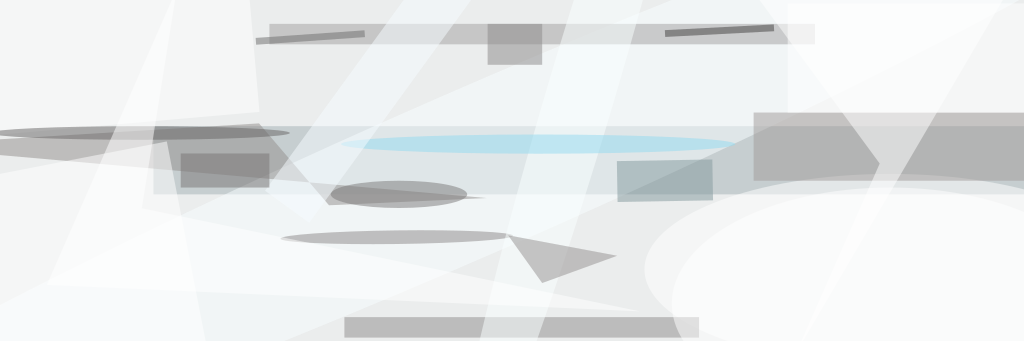A proposed method of compressing image data would exploit the well-known capability of a converging lens to generate the Fourier transform of an image by purely optical means, in much less time than is needed to compute the discrete Fourier transform of a sampled image by use of digital electronic circuits. Inasmuch as a transform (whether of the Fourier, discrete-cosine, or other type) is the most computation-intensive part of almost any electronic image-compression scheme, the speedup afforded by the proposed method could make the difference between success or failure in applications in which there are requirements to compress image data at high throughput rates. In addition, because high-speed digital image-processing circuits are typically power-hungry, the use of optical Fourier transformation can reduce power consumption.The Lens, Lasers, and Beam Splitters would be positioned to generate a hologram containing a Fourier transform of the input image. The optical propagation time to form the hologram would be much shorter than the time needed for digital electronic computation of the Fourier transform.

The Fourier-transform property of a converging lens can be summarized as follows: When the lens is placed at its focal distance from both an input and an output plane, then the image formed by the lens on the output plane is a Fourier transform of the object or image at the input plane. The two-dimensional spatial-frequency vector associated with any given point in the output image is proportional to the position vector from the optical axis to that point.

In the proposed method (see figure) the input image would be generated on a liquid-crystal spatial light modulator illuminated with a readout laser, which would be coherent with a reference laser. (It would be necessary to generate the input image in this way because the coherence of the laser light would be needed to form a hologram described subsequently.) A lens would be located at its focal distance from the input plane as well as from the output plane, where a charge-coupled-device (CCD) or an active-pixel sensor (APS) would be placed to detect the image. As a result, the Fourier transform of the input image would be formed on the image detector.

Capturing the intensity magnitude at the detector is not sufficient for reconstructing the image. For this reason, it would be necessary to write a hologram onto the image detector by means of interference between the lens-transformed image beam and the reference laser beam.

Because most of the information in a typical image is concentrated at low spatial frequencies, the bulk of information in optical Fourier transform would be concentrated about the optical axis. The image detector would sample the Fourier transform. The samples would be digitized, then entropy-coded by use of established digital electronic techniques

This work was done by Deborah Jackson of Caltech for NASA's Jet Propulsion Laboratory. For further information, access the Technical Support Package (TSP) free on-line at www.nasatech.com/tsp  under the Physical Sciences category.

NPO-20638

##### This Brief includes a Technical Support Package (TSP).